1. /
2. CBSE
3. /
4. Class 08
5. /
6. NCERT Solutions class-11 Chemistry...

# NCERT Solutions class-11 Chemistry Equilibrium### myCBSEguide App

Download the app to get CBSE Sample Papers 2023-24, NCERT Solutions (Revised), Most Important Questions, Previous Year Question Bank, Mock Tests, and Detailed Notes.

## NCERT Solutions class-11 Chemistry Equilibrium Part-2http://mycbseguide.com

26. Which of the following reactions will get affected by increasing the pressure?

Also, mention whether change will cause the reaction to go into forward or backward direction.

(i)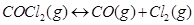(ii)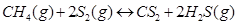(iii)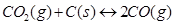(iv)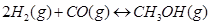(v)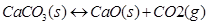(vi)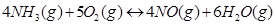27. The equilibrium constant for the following reaction is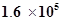at 1024 K.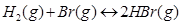Find the equilibrium pressure of all gases if 10.0 bar of HBr is introduced into a sealed container at 1024 K.

28. Dihydrogen gas is obtained from natural gas by partial oxidation with steam as per following endothermic reaction: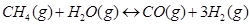(a) Write as expression for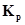for the above reaction.

(b) How will the values ofand composition of equilibrium mixture be affected by

(i) Increasing the pressure

(ii) Increasing the temperature

(iii) Using a catalyst?

29. Describe the effect of:

a) Addition of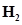b) Addition of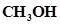c) Removal of CO

d) Removal ofon the equilibrium of the reaction:30. At 473 K, equilibrium constant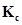for decomposition of phosphorus pentachloride,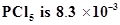. If decomposition is depicted as,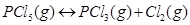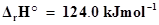a) Write an expression forfor the reaction.

b) What is the value offor the reverse reaction at the same temperature?

c) What would be the effect onif (i) more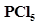is added (ii) pressure is increased? (iii) The temperature is increased?

31. Dihydrogen gas used in Haber’s process is produced by reacting methane from natural gas with high temperature steam. The first stage of two stage reaction involves the formation of CO and. In second stage, CO formed in first stage is reacted with more steam in water gas shift reaction,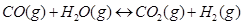If a reaction vessel at 400°C is charged with an equimolar mixture of CO and steam such that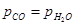4.0 bar, what will be the partial pressure ofat equilibrium?= 10.1 at 400°C

32. Predict which of the following reaction will have appreciable concentration of reactants and products:

a)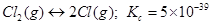b)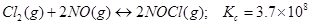c)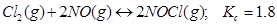33. The value offor the reaction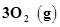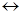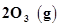is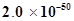at 25°C. If the equilibrium concentration of O2 in air at 25°C is 1.6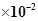, what is the concentration of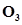?

34. The reaction, CO(g) +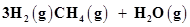is at equilibrium at 1300 K in a 1L flask. It also contain 0.30 mol of CO, 0.10 mol ofand 0.02 mol of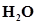and an unknown amount of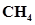in the flask. Determine the concentration ofin the mixture. The equilibrium constant,for the reaction at the given temperature is 3.90.

35. What is meant by the conjugate acid-base pair? Find the conjugate acid/base for the following species: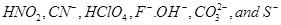36. Which of the followings are Lewis acids?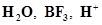, and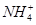37. What will be the conjugate bases for the Bronsted acids: HF,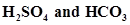?

38. Write the conjugate acids for the following Bronsted bases: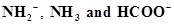.

39. The species:,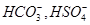, and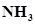can act both as Bronsted acids and bases. For each case give the corresponding conjugate acid and base.

40. Classify the following species into Lewis acids and Lewis bases and show how these act as Lewis acid/base: (a)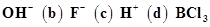.

41. The concentration of hydrogen ion in a sample of soft drink is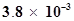M. what is its pH?

42. The pH of a sample of vinegar is 3.76. Calculate the concentration of hydrogen ion in it.

43. The ionization constant of HF, HCOOH and HCN at 298K are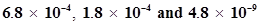respectively. Calculate the ionization constants of the corresponding conjugate base.

44. The ionization constant of phenol is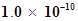. What is the concentration of phenolate ion in 0.05 M solution of phenol? What will be its degree of ionization if the solution is also 0.01M in sodium phenolate?

45. The first ionization constant of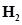­­S is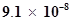. Calculate the concentration of HS- ion in its 0.1 M solution. How will this concentration be affected if the solution is 0.1 M in HCl also? If the second dissociation constant ofS is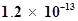, calculate the concentration of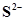under both conditions.

46. The ionization constant of acetic acid is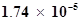. Calculate the degree of dissociation of acetic acid in its 0.05 M solution. Calculate the concentration of acetate ion in the solution and its pH.

47. It has been found that the pH of a 0.01M solution of an organic acid is 4.15. Calculate the concentration of the anion, the ionization constant of the acid and its pKa.

48. Assuming complete dissociation, calculate the pH of the following solutions:

(a) 0.003 M HCl

(b) 0.005 M NaOH

(c) 0.002 M HBr

(d) 0.002 M KOH

49. Calculate the pH of the following solutions:

a) 2 g of TlOH dissolved in water to give 2 litre of solution.

b) 0.3 g of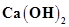dissolved in water to give 500 mL of solution.

c) 0.3 g of NaOH dissolved in water to give 200 mL of solution.

d) 1mL of 13.6 M HCl is diluted with water to give 1 litre of solution.

50. The degree of ionization of a 0.1M bromoacetic acid solution is 0.132. Calculate the pH of the solution and the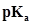of bromoacetic acid.### Test Generator

Create question paper PDF and online tests with your own name & logo in minutes.### myCBSEguide

Question Bank, Mock Tests, Exam Papers, NCERT Solutions, Sample Papers, Notes